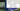# Longest Substring without repeating characters - Leet Code Solution

August 10, 2019## Problem Statement

Given a string, find the length of the longest substring without repeating characters.

``````Example 1:
Input: "abcabcbb"
Output: 3

Example 2:
Input: "bbbbb"
Output: 1

Example 3:
Input: "pwwkew"
Output: 3``````

## 1. Brute Force Solution

As the question demands substring. Why not we find out what are the substrings out of the input strings first. Lookout for the steps:

• Get all unique substrings. By unique I meant, there might be duplicate substrings as well. Count only unique one.
• Iterate over all substrings, and proceed for substring which have all unique characters
• Get the length of string
• Keep track of maximum length string

You got the answer. Lets look at the code:

``````public class SimpleSolution {
private String str;
public SimpleSolution(String str) {
this.str = str;
}

private Set<String> getAllUniqueSubstrings() {
Set<String> res = new HashSet<>();

int l = this.str.length();
for (int i=0; i<l; i++) {
for (int j=i+1; j<l; j++) {
}
}

return res;
}

//Checks if the string is having unique characters
private boolean isUniqueChars(String substring) {
Set<Character> chars = new HashSet<>();
int l = substring.length();

for (int i=0; i<l; i++) {
if (chars.contains(substring.charAt(i))) {
return false;
}
}
return true;
}

public String getResult() {
//Get all substrings
Set<String> substrings = this.getAllUniqueSubstrings();

//check their lengths, and return string with highest length
String result = null;
int maxLen = 0;
for (String substring : substrings) {
if (this.isUniqueChars(substring)) {
if (substring.length() > maxLen) {
result = substring;
maxLen = substring.length();
}
}
}

return result;
}
}``````

### Complexity

• Complexity on finding all substrings: O(n^2)
• Complexity on iterating over all substrings: O(n)
• Complexity on finding whether substring is having unique characters: O(n)

Overall complexity of this program is: O(n^2)

## 2. A Better Approach ~ O(n)

In above approach, our main time is in calculating substrings and checking if it has unique character. Lets have a look at the Sliding Window solution. We can maintain a HashSet to check if our substring has unique character or not. And, two variables for maintaining a sliding window.### Approach

• We have a HashSet to check unique characters in continuous substring
• We have two variables (i-start, j-end) to keep sliding window range.
• Iterate over string
• If the character is not found in HashSet, it will be added. And, sliding window end(variable j) will be incremented.
• In the meanwhile, keep track of maximum length unique string.
• If we found the character in HashSet, means we encounter a duplicate character. Remove the character from HashSet which is occuring as sliding window start (variable i) position.
• In a sliding window, we always have unique characters of a substring. And, it will be reduce to zero characters on encountering a duplicate character.

Lets look at the code:

``````public class OptimizedSolution {
private String str;

public OptimizedSolution(String str) {
this.str = str;
}

public String getResult() {
int l = this.str.length();
Set<Character> set = new HashSet<>();
int i=0;
int j=0;
String maxStr = "";

while (i < l && j < l) {
if (!set.contains(this.str.charAt(j))) {
j++;
String s = this.str.substring(i, j);
if (s.length() > maxStr.length()) {
maxStr = s;
}
}
else {
set.remove(this.str.charAt(i));
i++;
}
}

return maxStr;
}
}``````

### Complexity

Overall complexity of this program is: O(n) At the max, the program will run for O(2n) which is nearly equal to O(n)

## 3. Best Approach - O(n)

In above approach, we are moving left side of sliding window one by one on encountering a duplicate character. Our objective is to move that left pointer of sliding window to the occurance of duplicate character plus 1.Lets look at the code.

``````public class MoreOptimizedSolution {
private String str;
public MoreOptimizedSolution(String str) {
this.str = str;
}

public String getResult() {
Map<Character, Integer> map = new HashMap<>();
int l = this.str.length();
int i=0; int j=0;
String maxStr = "";

for (; j<l; j++) {
if (map.containsKey(this.str.charAt(j))) {
i = Math.max(map.get(this.str.charAt(j)), i);
}

if (j-i+1 > maxStr.length()) {
maxStr = this.str.substring(i, j+1);
}

map.put(this.str.charAt(j), j+1);
}

return maxStr;
}
}``````

### Complexity

The overall runtime of this code is: O(n), but is using extra space.